# Twenty Questions Subject Analytic Geometry Unit 2 Twenty

• Slides: 22Twenty Questions Subject: Analytic Geometry Unit 2Twenty Questions 1 2 3 4 5 6 7 8 9 10 11 12 13 14 15 16 17 18 19 20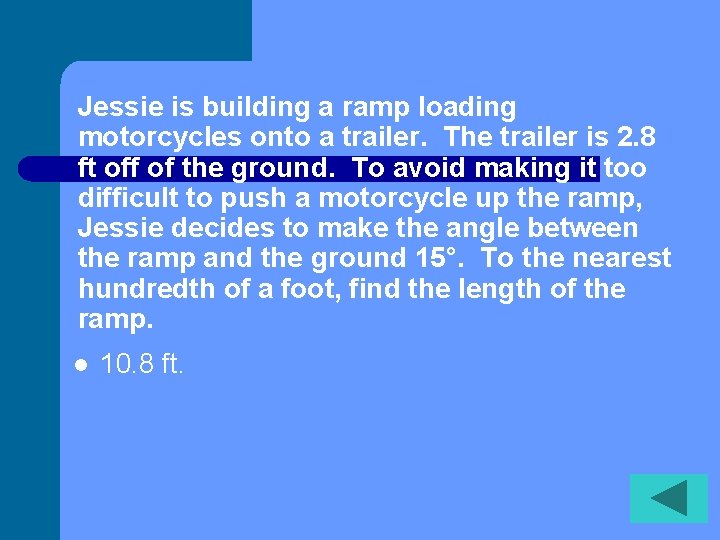Jessie is building a ramp loading motorcycles onto a trailer. The trailer is 2. 8 ft off of the ground. To avoid making it too difficult to push a motorcycle up the ramp, Jessie decides to make the angle between the ramp and the ground 15°. To the nearest hundredth of a foot, find the length of the ramp. l 10. 8 ft.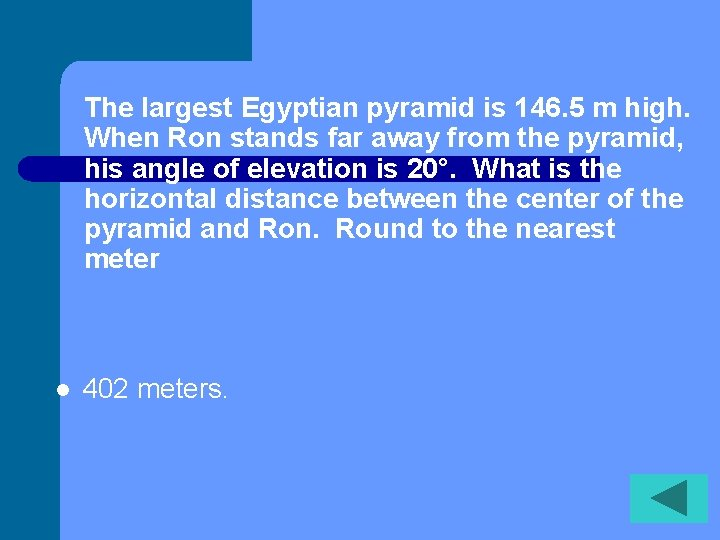The largest Egyptian pyramid is 146. 5 m high. When Ron stands far away from the pyramid, his angle of elevation is 20°. What is the horizontal distance between the center of the pyramid and Ron. Round to the nearest meter l 402 meters.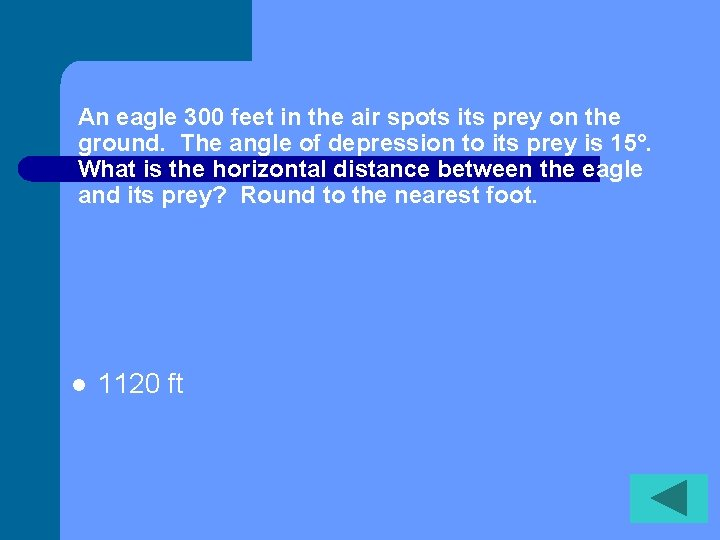An eagle 300 feet in the air spots its prey on the ground. The angle of depression to its prey is 15°. What is the horizontal distance between the eagle and its prey? Round to the nearest foot. l 1120 ftThe coordinates of the vertices of triangle RPQ are R(2, -1) P(2, 2) and Q(-2, -1). Find m∠P l 53°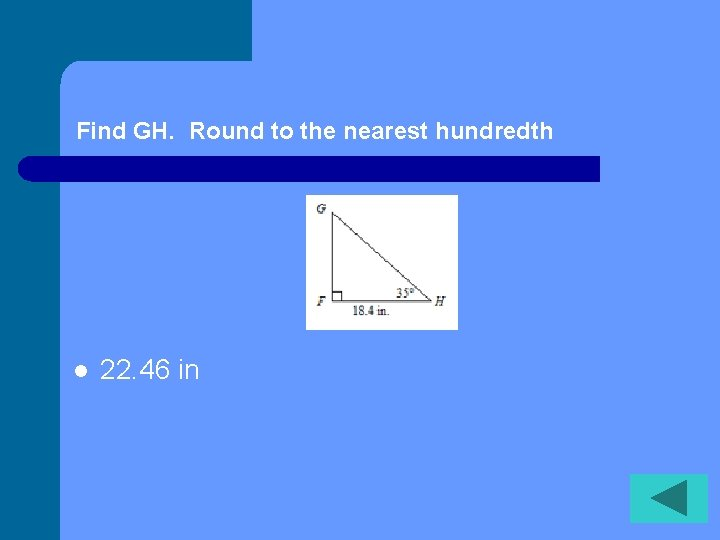Find GH. Round to the nearest hundredth l 22. 46 inThe size of a TV screen is given by the length of its diagonal. The screen aspect ratio is the ratio of its width to its height. The screen aspect ratio of a standard TV is 4: 3. What are the width and height of a 27” TV screen? l Width 21. 6 in height 16. 2 in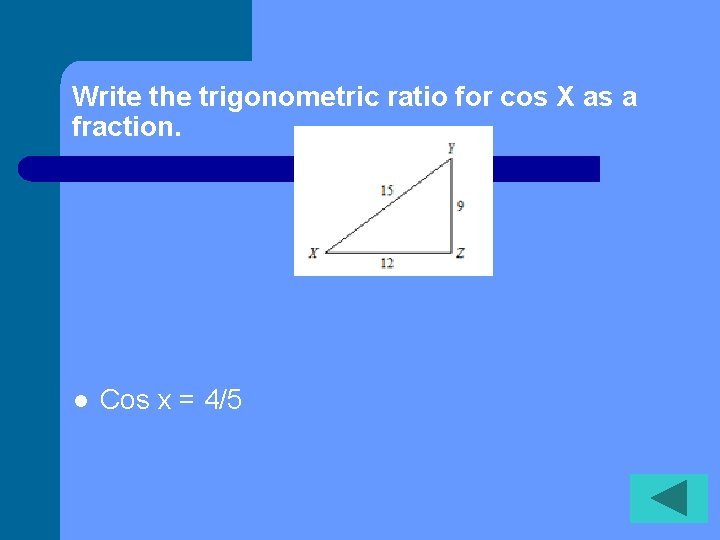Write the trigonometric ratio for cos X as a fraction. l Cos x = 4/5The longest side of a 30°-60°-90° triangle measures 8. What is the length of the longer leg? lWhen the angle of elevation of the sun is 50°, a flagpole casts a shadow that is 16. 8 ft long. What is the height of the flagpole to the nearest foot? . l 20 ftA utility worker is installing a 25 ft telephone pole. The work order indicates that two guy wires (a wire running from the ground to the top of the pole) should be placed opposite each other and at a 65° angle of elevation to the pole. To the nearest tenth of a foot, how far apart are the guy wires? l 23. 3 ft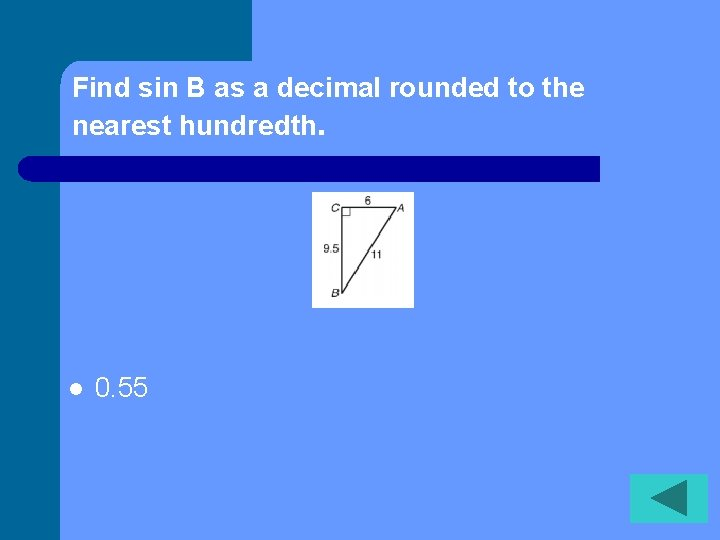Find sin B as a decimal rounded to the nearest hundredth. l 0. 55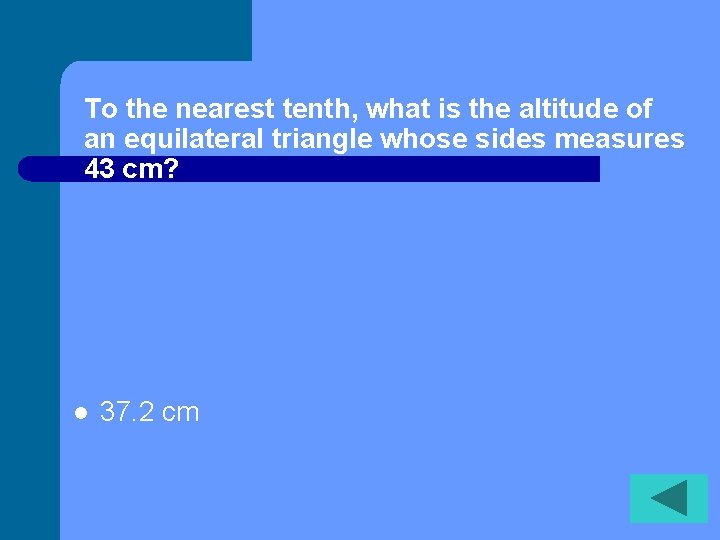To the nearest tenth, what is the altitude of an equilateral triangle whose sides measures 43 cm? l 37. 2 cm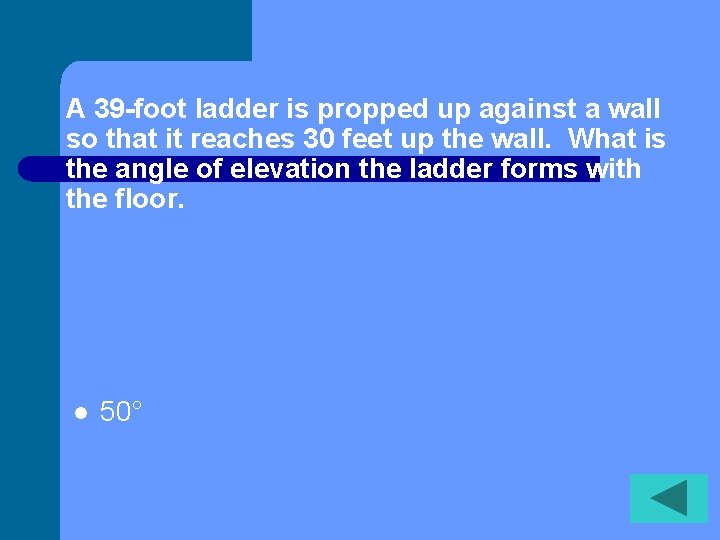A 39 -foot ladder is propped up against a wall so that it reaches 30 feet up the wall. What is the angle of elevation the ladder forms with the floor. l 50°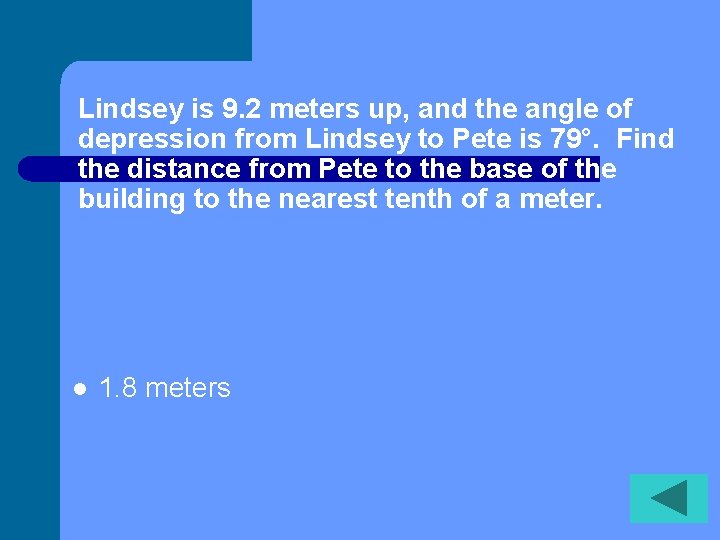Lindsey is 9. 2 meters up, and the angle of depression from Lindsey to Pete is 79°. Find the distance from Pete to the base of the building to the nearest tenth of a meter. l 1. 8 meters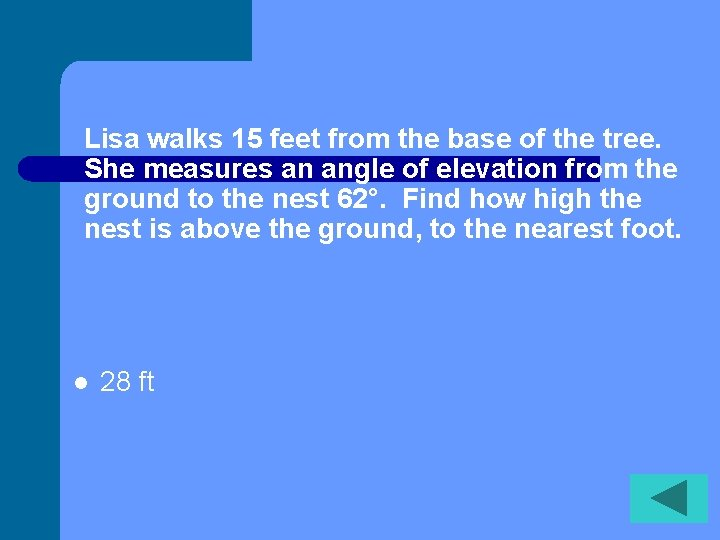Lisa walks 15 feet from the base of the tree. She measures an angle of elevation from the ground to the nest 62°. Find how high the nest is above the ground, to the nearest foot. l 28 ft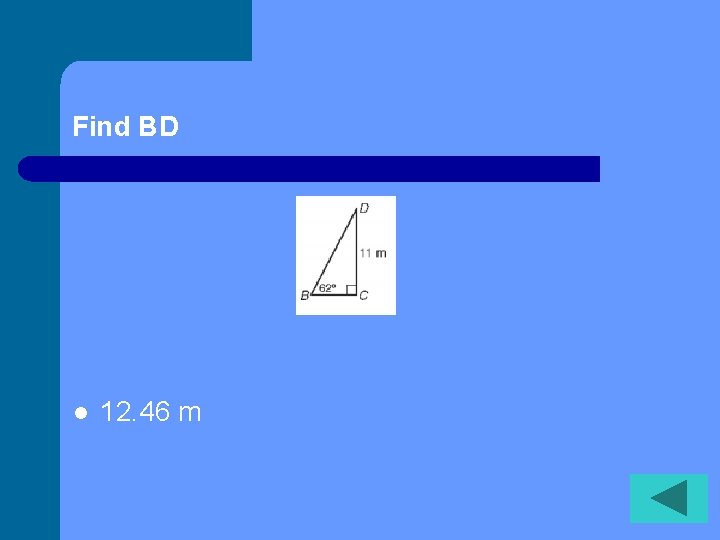Find BD l 12. 46 mFind QP l 19. 70 mm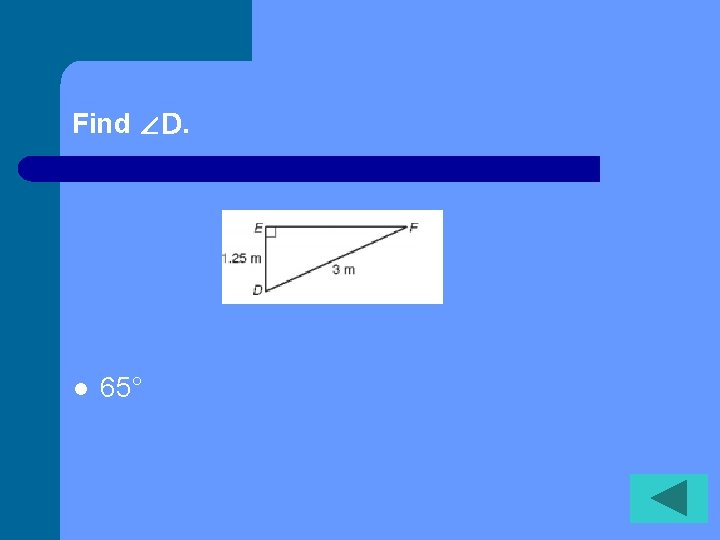Find ∠D. l 65°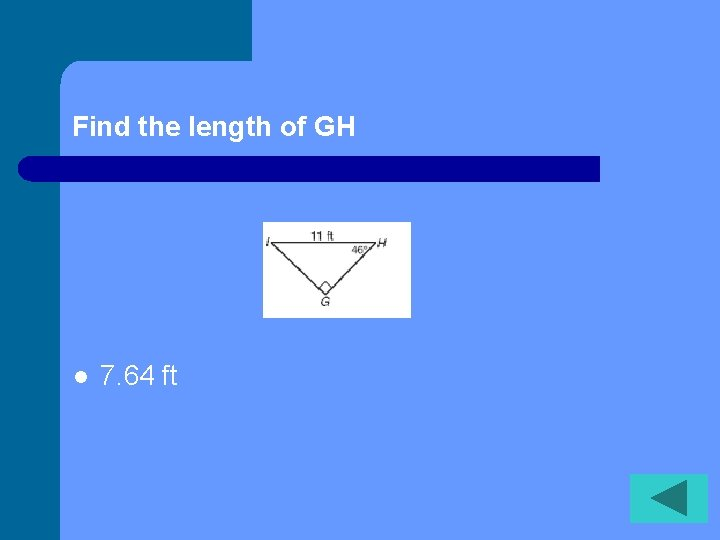Find the length of GH l 7. 64 ftA ramp is used to load a 4 -wheeler onto a truck bed that is 3 ft above the ground. The angle that the ramp makes with the ground is 32°. What is the horizontal distance covered by the ramp? Round to the nearest hundredth. l 4. 80 ft